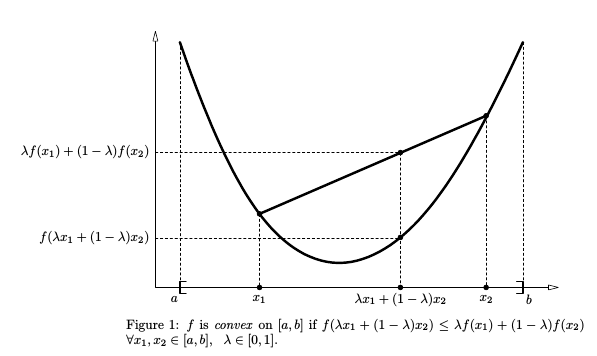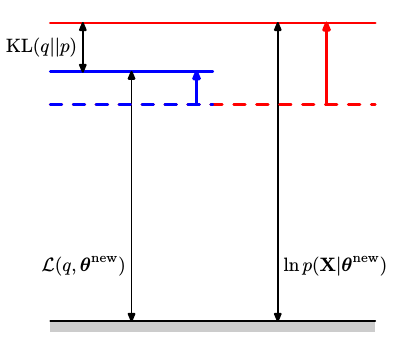# 机器学习：EM算法原理

EM算法是期望最大化 (Expectation Maximization) 算法的简称，用于含有隐变量的情况下，概率模型参数的极大似然估计或极大后验估计。EM算法是一种迭代算法，每次迭代由两步组成：E步，求期望 (expectation)，即利用当前估计的参数值来计算对数似然函数的期望值；M步，求极大 (maximization)，即求参数θθ 来极大化E步中的期望值，而求出的参数θθ将继续用于下一个E步中期望值的估计。EM算法在机器学习中应用广泛，本篇和下篇文章分别探讨EM算法的原理和其两大应用 —— K-means和高斯混合模型。

§1§1 先验知识

f(x)f(x)为定义在区间I=[a,b]I=[a,b]上的实值函数，对于任意x1,x2I,λ[0,1]∀x1,x2∈I,λ∈[0,1]，有：

f(λx1+(1λ)x2)λf(x1)+(1λ)f(x2)f(λx1+(1−λ)x2)≤λf(x1)+(1−λ)f(x2)

f(x)f(x)为凸函数 (convex function)，如下图所示。相应的，若上式中  变为  ，则f(x)f(x)为凹函数 (concave function)。 凸函数的判定条件是二阶导 f′′(x)0f″(x)⩾0，而凹函数为 f′′(x)0f″(x)⩽0 。后文要用到的对数函数ln(x)ln(x)的二阶导为1x2<0−1x2<0，所以是凹函数。Jensen不等式就是上式的推广，设f(x)f(x)为凸函数，λi0,iλi=1λi⩾0,∑iλi=1，则：

f(i=1nλixi)i=1nλif(xi)f(∑i=1nλixi)≤∑i=1nλif(xi)

ln(i=1nλixi)i=1nλiln(xi)ln(∑i=1nλixi)≥∑i=1nλiln(xi)

f(E[x])E[f(x)]f(E[x])≤E[f(x)]

KL散度

KL散度(Kullback-Leibler divergence) 又称相对熵 (relative entropy)，主要用于衡量两个概率分布p和q的差异，也可理解为两个分布对数差的期望。

KL(p||q)=ip(xi)logp(xi)q(xi)=Exp[logp(x)q(x)]=Exp[logp(x)logq(x)]KL(p||q)=∑ip(xi)logp(xi)q(xi)=Ex∼p[logp(x)q(x)]=Ex∼p[logp(x)−logq(x)]

KL散度总满足KL(p||q)0KL(p||q)⩾0，而当且仅当q=pq=p时，KL(p||q)=0KL(p||q)=0 。 一般来说分布p(x)p(x)比较复杂，因而希望用比较简单的q(x)q(x)去近似p(x)p(x)，而近似的标准就是KL散度越小越好。

KL散度不具备对称性，即KL(p||q)KL(q||p)KL(p||q)≠KL(q||p)，因此不能作为一个距离指标。

L(θ)=lnP(X|θ)L(θ)=lnP(X|θ)

P(θ|X)=P(X,θ)P(X)=P(θ)P(X|θ)P(X)P(θ)P(X|θ)P(θ|X)=P(X,θ)P(X)=P(θ)P(X|θ)P(X)∝P(θ)P(X|θ)

§2§2 EM算法初探

L(θ)=lnP(X|θ)=lnZP(X,Z|θ)(1.1)(1.1)L(θ)=lnP(X|θ)=ln∑ZP(X,Z|θ)

1. P(Z|X,θ(t))0P(Z|X,θ(t))⩾0
2. ZP(Z|X,θ(t))=1∑ZP(Z|X,θ(t))=1
3. ln()ln(⋅)为凹函数

L(θ)=lnZP(X,Z|θ)=lnZP(Z|X,θ(t))P(X,Z|θ)P(Z|X,θ(t))ZP(Z|X,θ(t))lnP(X,Z|θ)P(Z|X,θ(t))=ZP(Z|X,θ(t))lnP(X,Z|θ)Q(θ,θ(t))ZP(Z|X,θ(t))lnP(Z|X,θ(t))entropy(1.2)(1.3)(1.4)(1.2)L(θ)=ln∑ZP(X,Z|θ)=ln∑ZP(Z|X,θ(t))P(X,Z|θ)P(Z|X,θ(t))(1.3)⩾∑ZP(Z|X,θ(t))lnP(X,Z|θ)P(Z|X,θ(t))(1.4)=∑ZP(Z|X,θ(t))lnP(X,Z|θ)⏟Q(θ,θ(t))−∑ZP(Z|X,θ(t))lnP(Z|X,θ(t))⏟entropy

(1.3)(1.3)式构成了L(θ)L(θ)的下界，而(1.4)(1.4)式的右边为P(Z|X,θ(t))0P(Z|X,θ(t))的熵⩾0 ，其独立于我们想要优化的参数θθ，因而是一个常数。所以极大化L(θ)L(θ)的下界(1.3)(1.3)式就等价于极大化Q(θ,θ(t))Q(θ,θ(t))Q(θ,θ(t))Q(θ,θ(t)) (Q函数) 亦可表示为 EZ|X,θ(t)lnP(X,Z|θ)EZ|X,θ(t)lnP(X,Z|θ)，其完整定义如下：

===ZP(Z|X,θ(t))lnP(X,Z|θ(t))P(Z|X,θ(t))ZP(Z|X,θ(t))lnP(Z|X,θ(t))P(X|θ(t))P(Z|X,θ(t))ZP(Z|X,θ(t))lnP(X|θ(t))lnP(X|θ(t))=L(θ(t))∑ZP(Z|X,θ(t))lnP(X,Z|θ(t))P(Z|X,θ(t))=∑ZP(Z|X,θ(t))lnP(Z|X,θ(t))P(X|θ(t))P(Z|X,θ(t))=∑ZP(Z|X,θ(t))lnP(X|θ(t))=lnP(X|θ(t))=L(θ(t))

EM算法流程：

1. 初始化参数θ(0)θ(0)
2. E步： 利用当前参数θ(t)θ(t)计算Q函数， Q(θ,θ(t))=ZP(Z|X,θ(t))lnP(X,Z|θ)Q(θ,θ(t))=∑ZP(Z|X,θ(t))lnP(X,Z|θ)
3. M步： 极大化Q函数，求出相应的 θ=argmaxθQ(θ,θ(t))θ=argmaxθ⁡Q(θ,θ(t))
4. 重复 2. 和3. 步直至收敛。

EM算法也可用于极大后验估计，极大后验估计仅仅是在极大似然估计的基础上加上参数θθ的先验分布，即 p(θ)p(X|θ)p(θ)p(X|θ)，则取对数后变为lnp(X|θ)+lnp(θ)lnp(X|θ)+lnp(θ)，由于后面的lnp(θ)lnp(θ)不包含隐变量ZZ，所以E步中求Q函数的步骤不变。而在M步中需要求新的参数θθ，因此需要包含这一项，所以M步变为

θ=argmaxθ[Q(θ,θ(t))+ln(p(θ)]θ=argmaxθ⁡[Q(θ,θ(t))+ln(p(θ)]

§3§3 EM算法深入

L(θ)=lnP(X|θ)=Zq(Z)lnP(X|θ) q(Z)P(X,Z|θ)=Zq(Z)lnP(X|θ)q(Z)P(X,Z|θ)q(Z)P(X,Z|θ)=Zq(Z)P(X,Z|θ)q(Z)+Zq(Z)lnP(X|θ)q(Z)P(X,Z|θ)=Zq(Z)P(X,Z|θ)q(Z)+Zq(Z)lnq(Z)P(Z|X,θ)=Zq(Z)P(X,Z|θ)q(Z)L(q,θ)+KL(q(Z)||P(Z|X,θ)))(2.1)L(θ)=lnP(X|θ)=∑Zq(Z)lnP(X|θ)上下同乘以 q(Z)P(X,Z|θ)=∑Zq(Z)lnP(X|θ)q(Z)P(X,Z|θ)q(Z)P(X,Z|θ)=∑Zq(Z)P(X,Z|θ)q(Z)+∑Zq(Z)lnP(X|θ)q(Z)P(X,Z|θ)=∑Zq(Z)P(X,Z|θ)q(Z)+∑Zq(Z)lnq(Z)P(Z|X,θ)(2.1)=∑Zq(Z)P(X,Z|θ)q(Z)⏟L(q,θ)+KL(q(Z)||P(Z|X,θ)))

(2.1)(2.1)式的右端为q(Z)q(Z)和后验分布P(Z|X,θ)P(Z|X,θ)的KL散度，由此 lnP(X|θ)lnP(X|θ)被分解为L(q,θ)L(q,θ)KL(q||p)KL(q||p) 。由于KL散度总大于等于0，所以L(q,θ)L(q,θ)lnP(X|θ)lnP(X|θ)的下界，如图：L(θ) ，从而在隐变量存在的情况下实现了极大似然估计。在下一篇中将探讨EM算法的具体应用.